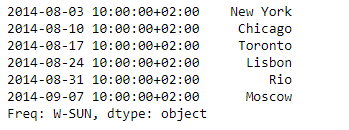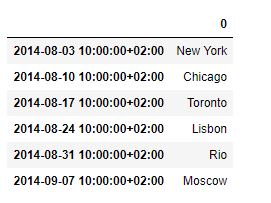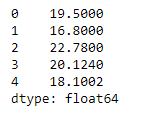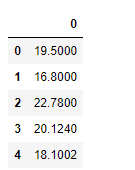Related Articles

# Python | Pandas Series.to_frame()

• Last Updated : 05 Feb, 2019

Pandas series is a One-dimensional ndarray with axis labels. The labels need not be unique but must be a hashable type. The object supports both integer- and label-based indexing and provides a host of methods for performing operations involving the index.

Pandas` Series.to_frame()` function is used to convert the given series object to a dataframe.

Syntax: Series.to_frame(name=None)

Parameter :
name : The passed name should substitute for the series name (if it has one).

Returns : data_frame : DataFrame

Example #1: Use `Series.to_frame()` function to convert the given series object to a dataframe.

 `# importing pandas as pd``import` `pandas as pd`` ` `# Creating the Series``sr ``=` `pd.Series([``'New York'``, ``'Chicago'``, ``'Toronto'``, ``'Lisbon'``, ``'Rio'``, ``'Moscow'``])`` ` `# Create the Datetime Index``didx ``=` `pd.DatetimeIndex(start ``=``'2014-08-01 10:00'``, freq ``=``'W'``, ``                     ``periods ``=` `6``, tz ``=` `'Europe/Berlin'``) `` ` `# set the index``sr.index ``=` `didx`` ` `# Print the series``print``(sr)`

Output :Now we will use `Series.to_frame()` function to convert the given series object to a dataframe.

 `# convert to dataframe``sr.to_frame()`

Output :As we can see in the output, the `Series.to_frame()` function has successfully converted the given series object to a dataframe.

Example #2: Use `Series.to_frame()` function to convert the given series object to a dataframe.

 `# importing pandas as pd``import` `pandas as pd`` ` `# Creating the Series``sr ``=` `pd.Series([``19.5``, ``16.8``, ``22.78``, ``20.124``, ``18.1002``])`` ` `# Print the series``print``(sr)`

Output :Now we will use `Series.to_frame()` function to convert the given series object to a dataframe.

 `# convert to dataframe``sr.to_frame()`

Output :As we can see in the output, the `Series.to_frame()` function has successfully converted the given series object to a dataframe.

Attention geek! Strengthen your foundations with the Python Programming Foundation Course and learn the basics.

To begin with, your interview preparations Enhance your Data Structures concepts with the Python DS Course. And to begin with your Machine Learning Journey, join the Machine Learning – Basic Level Course

My Personal Notes arrow_drop_up